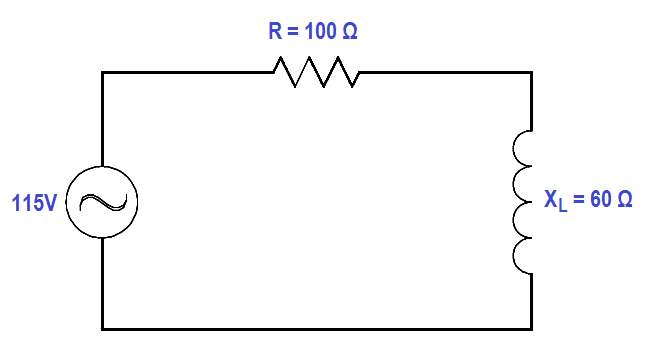# Impedance in RL Circuits

Impedance is the resultant of phasor addition of R and XL.

The symbol for impedance is Z.

Impedance is the total opposition to the flow of current and is expressed in ohms.

The below Equation is the mathematical representation of the impedance in an RL circuit.Example:

If a 100 Ω resistor and a 60 Ω Xare in series with a 115V applied voltage (Figure 6), what is the circuit impedance?Figure 6 : Simple R-L Circuit

Solution:Z = √ (1002 + 602)

Z = √ 13600

Z = 116.6 Ω

Don't Miss Our Updates
Be the first to get exclusive content straight to your email.
We promise not to spam you. You can unsubscribe at any time.